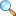Differential Geometry by Syed Hassan Waqas

These notes are provided and composed by Mr. Muzammil Tanveer. We are really very thankful to him for providing these notes and appreciates his effort to publish these notes on MathCity.org

 Name Differential Geometry Mr. Muzammil Tanveer 69 pages PDF (see Software section for PDF Reader) 2.7 MB

Contents & Summary

• Curvature
• Formula for Radius of Curvature
• Centre of Curvature
• Curvature and Radius of Curvature in Polar Form
• Space curve or Twisted curve or Skew curve
• Tangent line
• Normal line
• Secant line
• Equation of tangent of a point
• Normal plane
• Equation of Normal plane
• Oscillating plane or plane of Curvature
• Frenet-Serret Formula
• Torsion
• arameters other than ‘s’
• Equation of the Centre of Curvature
• Moving Trihedral
• Helix
• Spherical indicatrices
• Spherical Indicatrices of tangent
• Spherical Indicatrices of Normal
• Spherical Indicatrices of Binormal
• Surface
• Another definition of Surface
• Tangent plane
• Equation of Normal
• Parametric curves
• U-curves
• V-curves
• First order Fundamental magnitude
• Second order Fundamental form and second order Fundamental magnitude

Please click on View Online to see inside the PDF.

• Download PDF (2.64 MiB, 11091 downloads) |View Online